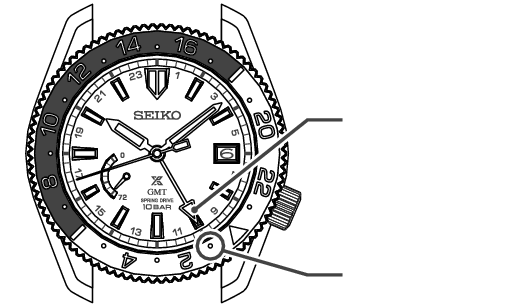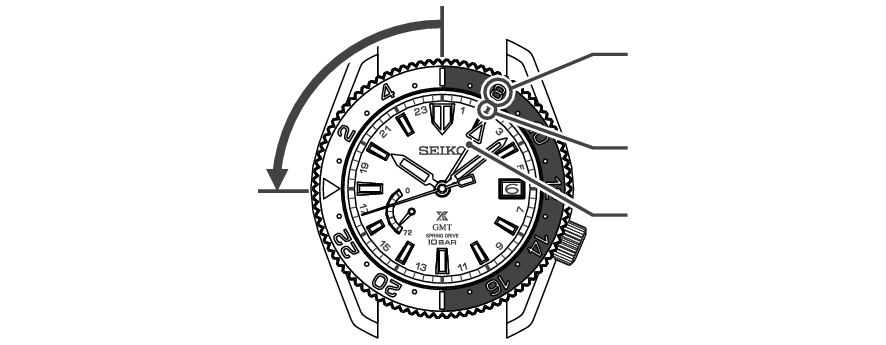> 5R66 (A model with a 24-hour hand) > How to use the rotating bezel with 24-hour scale (For models with rotating bezel having 24-hour scale)

# How to use the rotating bezel with 24-hour scale (For models with rotating bezel having 24-hour scale)

By turning the rotating bezel, another time can be read at the 24-hour hand.

## In the case of 24-hour hand being set to 24 hours of the hour and minute hands

<The direction and the amount to turn the rotating bezel can be determined by the following method>

[The direction and the amount to turn the rotating bezel] E
is calculated as,
[Time difference from UTC of 24-hour hand, C] - [Time difference from UTC of the region you wish to know, D]

E = C - D

In this example, the 24-hour hand indicates Japan time, and therefore, C = +9.

1. As an example, if the time of a region you wish to know belongs to the time zone of UTC, the time difference from UTC is “0” and hence,
D = 0
E = C - D = (+9) - (0) = +9
UTC can be read as “1:00” on the rotating bezel scale.

2. If E is a positive number “+”, turn the rotating bezel clockwise. If it is a negative number “-”, turn it counterclockwise.24-hour hand

1:00

3. As another example, if you wish to know the time of the region “Los Angeles”, the time difference from UTC is “-8 hours”, so D = -8
E = C - D = (+9) - (-8) = +17
Turn the rotating bezel 17 hours clockwise. (Result: Same as the counterclockwise rotation of 7 hours)
Los Angeles time can be read as “17:00”.

4. When this intended usage is no longer needed, please return the original position “24” mark of rotating bezel to 12 o’clock position.24-hour hand

17:00

## In the case of 24-hour hand being set to different time zone

Three different time zones can be read by turning the rotating bezel.

【Example】 When displaying 10:08 AM Japan time with the hour and minute hands, and displaying Paris time with the 24-hour hand,

Time difference from UTC of “Paris” where the 24-hour hand is set, C = +1
Time difference from UTC of “Bangkok” that you wish know from now on, D = +7
E=C-D= (+1) - (+7) = -6
Turn the rotating bezel counterclockwise and set.24-hour hand

Bangkok
“8 AM”

Paris
“2 AM”

Turn counterclockwise for 6 gradations in
24-hour format:
6 hours minus